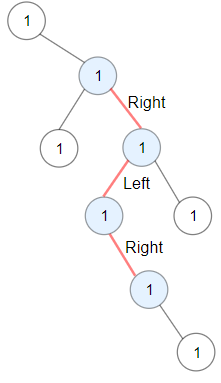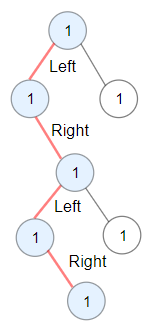1372. Longest ZigZag Path in a Binary Tree

Medium

Given a binary tree root, a ZigZag path for a binary tree is defined as follow:

• Choose any node in the binary tree and a direction (right or left).
• If the current direction is right then move to the right child of the current node otherwise move to the left child.
• Change the direction from right to left or right to left.
• Repeat the second and third step until you can’t move in the tree.

Zigzag length is defined as the number of nodes visited - 1. (A single node has a length of 0).
Return the longest **ZigZag** path contained in that tree.#### Example 1:

Input: root = [1,null,1,1,1,null,null,1,1,null,1,null,null,null,1,null,1]
Output: 3
Explanation: Longest ZigZag path in blue nodes (right -> left -> right).#### Example 2:

Input: root = [1,1,1,null,1,null,null,1,1,null,1]
Output: 4
Explanation: Longest ZigZag path in blue nodes (left -> right -> left -> right).


#### Example 3:

Input: root = 
Output: 0


#### Constraints:

• Each tree has at most 50000 nodes..
• Each node's value is between [1, 100]
• .

#### JavaScript Solution

/**
* Definition for a binary tree node.
* function TreeNode(val) {
*     this.val = val;
*     this.left = this.right = null;
* }
*/
/**
* @param {TreeNode} root
* @return {number}
*/
var longestZigZag = function (root) {};

••